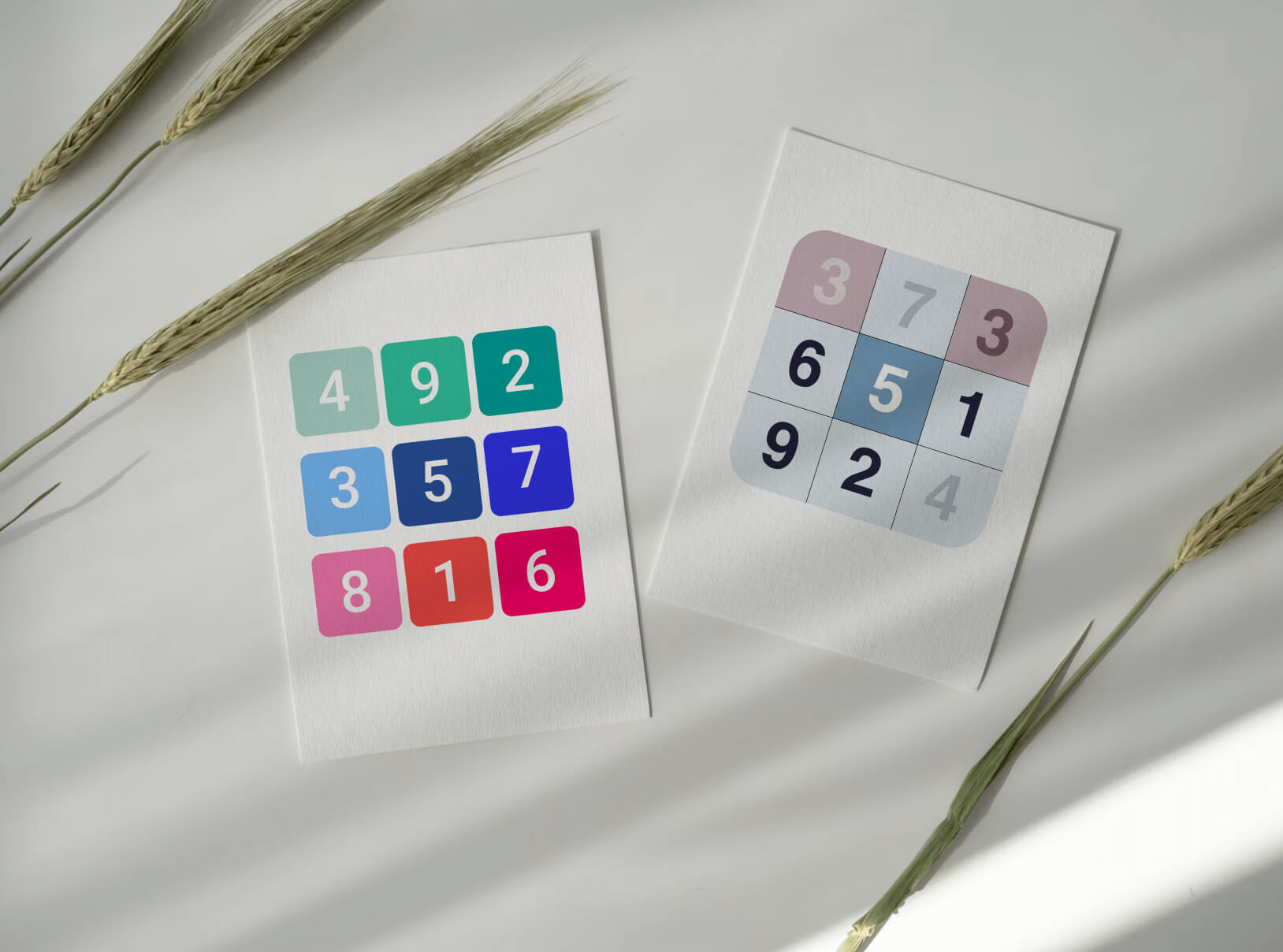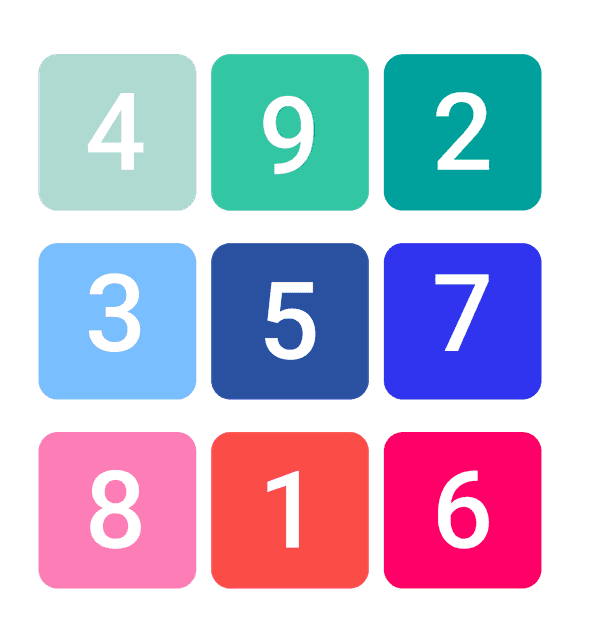### Magic Squares: A Fascinating Puzzle of Numbers

Magic squares are an intriguing mathematical concept that have been studied and admired for centuries. A magic square is a grid of numbers arranged in such a way that the sum of the numbers in each row, column, and diagonal is the same. This sum is called the "magic sum" or "magic constant" of the square.The origins of magic squares can be traced back to ancient China and India, where they were used for divination and other mystical practices. Over time, magic squares became a subject of mathematical study and have been explored by many famous mathematicians throughout history.

Magic squares come in many different sizes and orders, with the number of cells in each row and column determining the order of the square. The simplest magic square is the 3x3 square, also known as the "Lo Shu Square," while larger magic squares can have orders as high as several thousand.

Despite their seemingly simple rules, magic squares have numerous intriguing properties and applications, making them a fascinating subject for study and exploration. In the following sections, we will explore the different types of magic squares, their properties, famous examples, and real-world applications.

## Types of Magic Squares

Magic squares come in different types based on the order of the square and the numbers used in the grid. Here are the five main types of magic squares:

### 1. Odd-order magic squares

Odd-order magic squares have an odd number of cells in each row and column. The most common type of odd-order magic square is the 3x3 square, also known as the Lo Shu Square. In an odd-order magic square, the magic sum is calculated as:

``````M = (n^2 + 1) / 2
``````

where `n` is the order of the square.

### 2. Even-order magic squares

Even-order magic squares have an even number of cells in each row and column. The most common type of even-order magic square is the 4x4 square. Unlike odd-order magic squares, even-order magic squares cannot be constructed using the simple method of adding consecutive numbers. Instead, they require more complex methods such as the LUX method or the Siamese method.

### 3. Composite magic squares

Composite magic squares are made up of smaller magic squares arranged in a specific pattern to form a larger magic square. These types of magic squares were popularized by Albrecht Dürer's famous "Melencolia I" engraving.

### 4. Prime magic squares

Prime magic squares use only prime numbers in their grid. These types of magic squares are rare and have unique properties. In prime magic squares, the magic sum can be calculated as:

``````M = n * (n^2 + 1) / 2
``````

where `n` is the order of the square.

### 5. Diabolic magic squares

Diabolic magic squares have the additional property that every broken diagonal also sums to the magic sum. These types of magic squares were first introduced by the mathematician Gaston Tarry in 1901.

Each type of magic square has its own unique properties and characteristics, making them all the more fascinating and intriguing to study.

For more information on the different types of magic squares, check out this source.

## Properties of Magic Squares

Magic squares have some interesting properties that make them unique and intriguing. Here are some of the key properties of magic squares:

### 1. Symmetry

Magic squares have various types of symmetry depending on the order of the square. For example, odd-order magic squares have rotational symmetry, while even-order magic squares have reflectional symmetry.

### 2. Magic Constant

The magic constant is the sum of each row, column, and diagonal in a magic square. In an `n x n` magic square, the magic constant is `M = n * (n^2 + 1) / 2`. The magic constant is the same regardless of the numbers used in the square.

### 3. Unique Numbers

Each number in a magic square is unique, and no number is repeated. This property is what makes constructing magic squares a challenging and intriguing puzzle.

### 4. Magic Property

The most significant property of a magic square is that each row, column, and diagonal adds up to the magic constant. This is what gives magic squares their name and makes them so fascinating.

### 5. Transposition Property

Transposing a magic square (i.e., switching its rows and columns) results in another magic square with the same magic constant. This property is unique to magic squares and is not found in regular squares.

### 6. Associated Squares

A magic square can have up to eight associated squares that also share the magic constant. These associated squares are obtained by rotating and reflecting the original square.

Magic squares are not only fascinating for their aesthetic beauty but also for their unique and intriguing properties. These properties make magic squares an intriguing puzzle for mathematicians, artists, and puzzle enthusiasts alike.

For more information on the properties of magic squares, check out this source.

## Famous Magic Squares

Throughout history, several famous magic squares have been discovered, each with its unique properties and history. Here are some of the most well-known magic squares:

### 1. Lo Shu Square

The Lo Shu Square is the oldest known magic square, dating back to ancient China. It is a `3 x 3` magic square with the numbers 1 to 9 arranged such that each row, column, and diagonal adds up to 15.

### 2. Albrecht Dürer's Magic Square

Albrecht Dürer, a German artist, and mathematician, created a famous magic square in 1514. The magic square is a `4 x 4` square that contains the numbers 1 to 16 arranged such that each row, column, and diagonal adds up to 34.

### 3. Benjamin Franklin's Magic Square

Benjamin Franklin, one of the founding fathers of the United States, also created a famous magic square. His magic square is a `8 x 8` square that contains the numbers 1 to 64 arranged such that each row, column, and diagonal adds up to 260.

### 4. The Siamese Method

The Siamese Method is a technique for constructing magic squares invented by a Thai mathematician named Narayana in the 14th century. The method involves starting with the number 1 in the middle of the square and then filling in the rest of the numbers in a specific pattern.

### 5. Franklin's Squares

Franklin's Squares are a series of magic squares created by Benjamin Franklin. They are unique in that they are not only magic squares but also contain many other interesting properties, such as palindromic diagonals and corners that add up to the magic constant.

These are just a few of the many famous magic squares that have been discovered throughout history. Each square has its unique story and properties that make it fascinating to mathematicians, artists, and puzzle enthusiasts alike.

## Applications of Magic Squares

Magic squares have been studied and appreciated for their beauty and mathematical properties for centuries. But beyond their academic value, they also have practical applications in various fields. Here are some of the most common applications of magic squares:

### 1. Recreational Puzzles

One of the most popular applications of magic squares is in creating recreational puzzles. Magic squares can be used as the basis for a wide range of puzzles, from simple ones for children to complex ones for adults. These puzzles often involve finding the missing numbers in a partially filled magic square or creating a magic square that meets specific criteria.

### 2. Encryption and Coding

Magic squares can also be used in encryption and coding applications. By assigning letters or symbols to the numbers in a magic square, one can create a code that is difficult to crack without the knowledge of the specific magic square used. This technique has been used in various historical and modern cryptography systems.

### 3. Art and Design

Magic squares have been used as a basis for artistic designs and patterns throughout history. In particular, Islamic art and architecture have many examples of magic squares incorporated into their designs. The use of magic squares in art and design demonstrates the beauty and symmetry inherent in these mathematical objects.

### 4. Divination and Superstition

Magic squares have also been used in various forms of divination and superst

## Conclusion

In conclusion, magic squares are fascinating mathematical objects that have been studied for centuries. They have many interesting properties and applications, ranging from recreational puzzles to encryption and coding, art and design, and even divination and superstition. The study of magic squares is a great way to develop one's mathematical intuition and problem-solving skills.

Whether you are a mathematician, puzzle enthusiast, artist, or simply someone who appreciates the beauty and elegance of mathematics, magic squares have something to offer. By exploring the different types, properties, and applications of magic squares, you can gain a deeper appreciation for the power and versatility of mathematics.